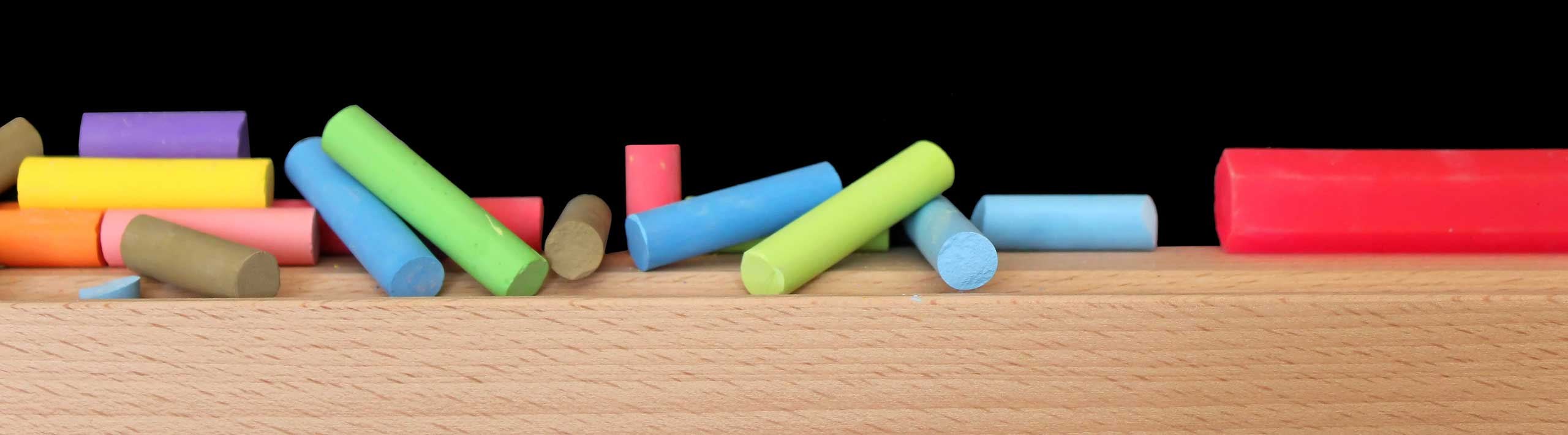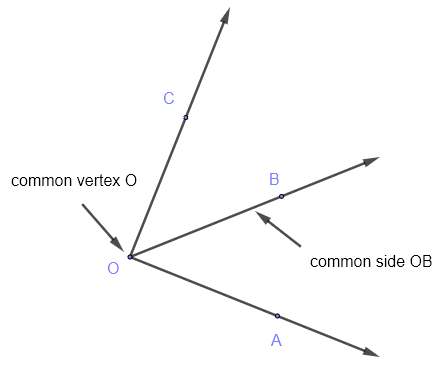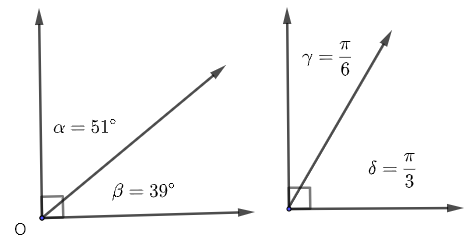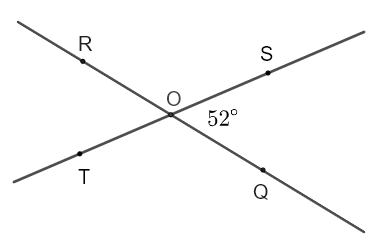# Angles in Geometry

Definitions and properties of angles in geometry are presented along with questions and solutions.

## An Angle in Geometry

An angle is formed when two rays intersect at a common point $O$ called the vertex of the angle  . An angle is also a mesure of the rotation required to superimpose one of two intersecting rays, ray 1 for example, on the other ray, ray 2 for example.
The rays forming an angle are also called sides of the angle.
Examples of angles are shown in the figure below.## Units of Angle Measures

1) Angles are measured in degrees $(\; ^{\circ} \;)$.
Example: $\alpha = 60^{\circ}$
2) Angles are also measured in radians but the radian unit is not mentioned because it is dimensionless.
Example: $\beta = 2.3$ , $\gamma = \dfrac{2 \pi}{5}$.
Note that the unit of angles $\beta$ and $\gamma$ above are assumed to be radians.

## Identification and Denotation of Angles

Any of the Greek letters such $\alpha, \beta, \gamma$ ... may be used as a name and a size of an angle. Other latin letters, such as $A, a, B, b .. .$ may also be used.
It is also common practice to use the vertex and points on the side of the angle to denote an angle as follows: $\angle AOB$.
The measure (or size) of an angle with the above denotation is as follows: For example $m \angle AOB = 23^{\circ}$Two angles are adjacent if they have a common side and common vertex. In the figure below angles $\angle AOB$ and $\angle BOC$ have a common side $OB$ and common vertex $O$ are therefore adjacent.Note that $m \angle AOC = m \angle AOB + m \angle BOC$

## Complete Angle

If starting from ray 1, ray 2 is rotated all the way and then superimposed to ray 1 making one full rotation, it makes a complete angle whose measure is by definition of $360^{\circ}$ or $2 \pi$ radians.## Right Angle

A right angle is an angle with measure equal to $90^{\circ}$ or $\dfrac{\pi}{2}$ radians.Notes
1) The small square at the vertex indicating a right angle.
2) Two lines that intersect and make an angle of $90^{\circ}$ are called
perpendicular lines .

## Straight Angle

A straight angle is an angle with measure equal to $180^{\circ}$ or $\pi$ radians.## Acute Angle

An acute angle is an angle with a measure between $0$ and $90^{\circ}$ or between $0$ and $\dfrac{\pi}{2}$ radians.## Obtuse Angle

An obtuse angle is an angle with a measure between $90$ and $180^{\circ}$ or between $\dfrac{\pi}{2}$ and $\pi$ radians.## Complementary Angles

Two angles are complementary if they share one common side and the sum of their measures is equal to $90^{\circ}$ or $\dfrac{\pi}{2}$.Examples:
1) Angles $\alpha$ and $\beta$ with measures $\alpha = 51^{\circ}$ and $\beta = 39^{\circ}$ are complementary since $\alpha + \beta = 90^{\circ}$

2) Angles $\gamma$ and $\delta$ with measures $\gamma = \dfrac{\pi}{6}$ and $\delta = \dfrac{\pi}{6}$ are complementary since $\gamma +\delta = \dfrac{\pi}{2}$

## Supplementary Angles

Two angles are supplementary if they share one common side and the sum of their measures is equal to $180^{\circ}$ or $\pi$.Examples:
1) Angles $\gamma$ and $\delta$ with measures $\gamma = \dfrac{\pi}{6}$ and $\delta = \dfrac{5 \pi}{6}$ are supplementary since $\gamma +\delta = \pi$

2) Angles $\alpha$ and $\beta$ with measures $\alpha = 127^{\circ}$ and $\beta = 53^{\circ}$ are supplementary since $\alpha + \beta = 180^{\circ}$

## Vertical Angles

Vertical angles are formed by the intersection of two straight lines. In the figure below angles $\angle COA$ and $\angle DOB$ are vertical angles. Angles $\angle AOD$ and $\angle BOC$ are also vertical anglesVertical angles are congruent. Hence $m\angle COA = m\angle DOB$ and $m\angle AOD = m\angle BOC$

## Convert Angle From Degrees to Radians

Let $\theta$ be an angle in degrees.
In radians, $\theta$ is given by: $\Large{\color{red}{\dfrac{\theta \times \pi}{180}}}$ Example: Convert $\theta = 120^{\circ}$ into radians. $\dfrac{120 \times \pi}{180} = \dfrac{2 \pi}{3} \approx 2.09439$

## Convert Angle From Radians to Degrees

Let $\alpha$ be an angle in radians.
In degrees, $\alpha$ is given by: $\Large{\color{red}{ \dfrac{\alpha \times 180}{\pi} }}$ Example 1:
Convert $\theta = 2.1$ into degrees.
Solution $\dfrac{2.1 \times 180}{\pi} = \dfrac{378}{\pi} \approx 120.32113^{\circ}$ Example 2:
Convert $\theta = \dfrac{3 \pi}{4}$ into degrees.
Solution $\dfrac{\dfrac{3 \pi}{4} \times 180}{\pi} = 135^{\circ}$

## Special Angles in Degrees and Radians

 Angle in Degrees Angle in Radians $0^{\circ}$ $0$ $30^{\circ}$ $\dfrac{\pi}{6}$ $60^{\circ}$ $\dfrac{\pi}{3}$ $90^{\circ}$ $\dfrac{\pi}{2}$ $120^{\circ}$ $\dfrac{2\pi}{3}$ $150^{\circ}$ $\dfrac{5\pi}{6}$ $180^{\circ}$ $\pi$ $210^{\circ}$ $\dfrac{7\pi}{6}$ $240^{\circ}$ $\dfrac{4\pi}{3}$ $270^{\circ}$ $\dfrac{3\pi}{2}$ $300^{\circ}$ $\dfrac{5\pi}{3}$ $330^{\circ}$ $\dfrac{11\pi}{6}$ $360^{\circ}$ $2 \pi$

## Questions

(See solutions below)

### Part A

Given angles $a, b, c, d, e$ and $f$ with measures
$a = 21^{\circ}$
$b = 90.1^{\circ}$
$c = 90^{\circ}$
$d = 134.2^{\circ}$
$e = 69^{\circ}$
$f = 45.8^{\circ}$

1. Which of the above angles are acute?
2. Which of the above angles are obtuse?
3. Which pairs of the above angles are complementary?
4. Which pairs of the above angles are supplementary?

### Part B

1. $A$ and $B$ are complementary angles such that $A = 22^{\circ}$. Find the measure of angle $B$
2. $\alpha$ and $\beta$ are supplementary angles such that $\alpha = 0.5 \pi$. Find the measure of angle $\beta$
3. $a$ and $b$ are supplementary angles such that $b = 2 a$. Find the measures of angles $a$ and $b$ in degrees.
4. $C$ and $D$ are complementary angles such that $D - C = 23^{\circ}$. Find the measures of angles $C$ and $D$
5. Given the measure of angle $\angle QOS = 52^{\circ}$, find the measures of angles $\angle ROT$ and $\angle SOR$ in the figure below.6. Given the measure of angle $\angle AOC = 90^{\circ} , \angle AOB = 67^{\circ}$ and $\angle GOH = 27^{\circ}$, find the measure of angles $\angle DOF$ in the figure below.### Part C

1. Convert the angles $A = 22^{\circ} , B = 145^{\circ}$ and $C = 90^{\circ}$ into radians.
2. Convert the angles $a = 0.2 , b = 2.5$ and $c = \dfrac{\pi}{3}$ into degrees.

## Solutions

### Part A

1. Angles in parts $a, e$ and $f$ are acute since each one of these angles is between $0$ and $90^{\circ}$.
2. Angles in parts $b$ and $d$ are obtuse since each one of these angles is between $90$ and $180^{\circ}$.
3. Angles in parts $a$ and $e$ are complementary since $a + e = 90^{\circ}$
4. Angles in parts $d$ and $f$ are supplementary since $d + f = 180^{\circ}$

### Part B

1. $A$ and $B$ are complementary angles, hence $\quad A + B = 90^{\circ}$.
Substitute $A$ by its value in the above equation. $\quad 22^{\circ} + B = 90^{\circ}$
Solve for $B$: $\quad B = 90^{\circ} - 22^{\circ} = 68^{\circ}$

2. $\alpha$ and $\beta$ are supplementary, hence $\quad \alpha + \beta = \pi$
Substitute $\alpha$ by its value in the above equation: $\quad 0.5 \pi + \beta = \pi$
Solve for $\beta$: $\quad \beta = \pi - 0.5 \pi = 0.5 \pi$

3. $a$ and $b$ are supplementary angles, hence $\quad a + b = 180^{\circ}$
Substitute $b$ by $2 a$ in the above equation: $\quad a + 2a = 180^{\circ}$
Group like terms: $\quad 3 a = 180^{\circ}$
Solve for $a$: $\quad a = \dfrac{180^{\circ}}{3} = 60 ^{\circ}$
$\quad b = 2 a = 2 \times 60 ^{\circ} = 120^{\circ}$

4. $C$ and $D$ are complementary angles, hence $\quad C + D = 90^{\circ}$
Given that $D - C = 23^{\circ}$, we may write: $\quad D = 23^{\circ} + C$
Substitute $D$ by $23^{\circ} + C$ in the equation $\quad C + D = 90^{\circ}$ to obtain: $\quad C + 23^{\circ} + C = 90^{\circ}$
Group like term: $\quad 2 C = 67^{\circ}$
Solve for $C$ : $\quad C = 33.5^{\circ}$
$\quad D = 23^{\circ} + C = 23^{\circ} + 33.5^{\circ} = 55.5^{\circ}$

5. $\angle QOS$ and $\angle ROT$ are vertical angles and therefore congruent. Hence, $\quad m \angle ROT = m\angle QOS = 52^{\circ}$
$\angle SOR$ and $\angle ROT$ are supplementary angles and therefore: $\quad m\angle SOR + m\angle ROT = 180^{\circ}$
Substitute $m \angle ROT$ by its value found above, we obtain: $\quad m\angle SOR + 52^{\circ} = 180^{\circ}$
Solve for $\quad m\angle SOR$: $m\angle SOR = 180^{\circ} - 52^{\circ} = 128^{\circ}$6. Since $m\angle AOC = 90^{\circ}$, the lines through $C , G$ and $E , A$ are perpendicular and therofore $\quad m \angle COE = m \angle EOG = m \angle GOA = 90^{\circ}$
Angles $\angle GOH$ and $\angle COD$ are vertical and therefore congruent; hence $\quad m\angle COD = 27^{\circ}$
$\angle COD$ and $\angle DOE$ are complementary angles and therefore $\quad m\angle COD + m\angle DOE = 90^{\circ}$
Substitute $m\angle COD$ by its value found above: $\quad 27^{\circ} + m\angle DOE = 90^{\circ}$
Solve for $m\angle DOE$: $\quad m\angle DOE = 63^{\circ}$
$\angle EOF$ and $\angle AOB$ are vertical angles and therefore congruent. Hence, $\quad m \angle EOF = m\angle AOB = 67^{\circ}$
Angles $\angle DOE$ and $\angle EOF$ are adjacent (have a common side OE), we therefore write that: $\quad m\angle DOF = m\angle DOE + m\angle EOF = 63^{\circ} + 67^{\circ} = 130^{\circ}$Note that there are many other ways to find the measure of $\angle DOF$.

### Part C

1. Angle $A = 22^{\circ}$ in radians is given by: $\quad \dfrac{ A \times \pi}{180} = \dfrac{22 \times \pi}{180} = \dfrac{11\pi }{90} \approx 0.38397$

Angle $B = 145^{\circ}$ in radians is given by: $\quad \dfrac{ B \times \pi}{180} = \dfrac{145 \times \pi}{180} = \dfrac{29\pi }{36} \approx 2.53072$

Angle $C = 90^{\circ}$ in radians is given by: $\quad \dfrac{ C \times \pi}{180} = \dfrac{90 \times \pi}{180} = \dfrac{\pi }{2} \approx 1.57079$

Convert angles into degrees
2. Angle $a = 0.2$ in degrees is given by: $\quad \dfrac{ a \times 180}{\pi} = \dfrac{ 0.2 \times 180}{\pi} = \dfrac{36}{\pi } \approx 11.45915^{\circ}$

Angle $b = 2.5$ in degrees is given by: $\quad \dfrac{ b \times 180}{\pi} = \dfrac{ 2.5 \times 180}{\pi} = \dfrac{450}{\pi } \approx 143.23944^{\circ}$

Angle $c = \dfrac{\pi}{3}$ in degrees is given by: $\quad \dfrac{ c \times 180}{\pi} = \dfrac{ \dfrac{\pi}{3} \times 180}{\pi} = 60^{\circ}$

## More References and Links to Geometry Tutorials

Questions on Angles with Solutions and Explanations
Geometry Tutorials and Problems
The Four Pillars of Geometry - John Stillwell - Springer; 2005th edition (Aug. 9 2005) - ISBN-10 : 0387255303
Geometry: A Comprehensive Course - Daniel Pedoe - Dover Publications - 2013 - ISBN: 9780486131733